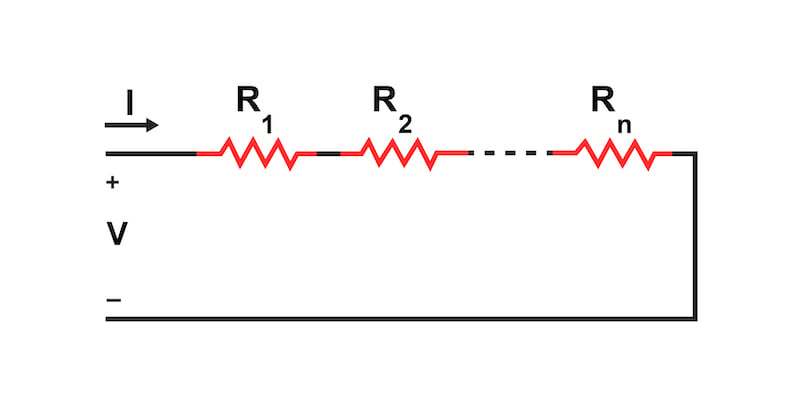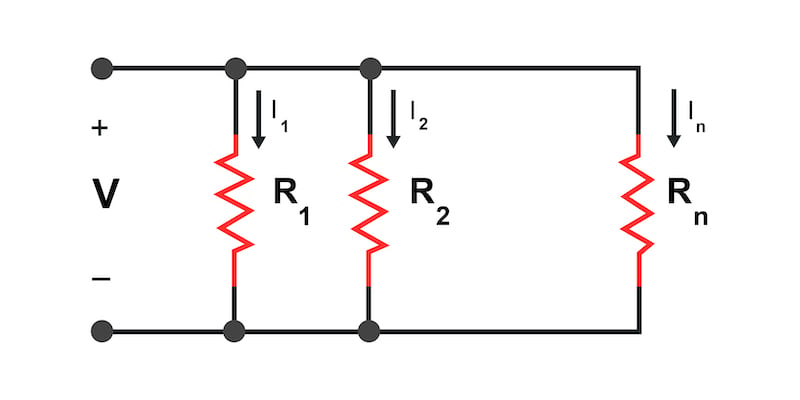# Power Dissipation Calculator for Series and Parallel Circuits

## This tool will calculate the power dissipation of series or parallel resistor circuits given the input voltage and the resistance values.

Inputs
Connection Type:
Voltage:
R - {{ key + 1}} X

Outputs
P - {{ key + 1}}

### Calculator Operation

1. Select either series or parallel for the type of resistive circuit
2. Enter the source voltage
3. Enter a resistance value for at least one resistor
5. Remove resistors by pushing the "x" button to the left of the value box
6. Push the calculate button to determine the power dissipated by each resistor

### Series Circuit Schematic and Power Equations

Figure 1 is the circuit schematic for resistors connected in series with an applied voltage V.##### Figure 1. Resistive series circuit schematic for power dissipation calculation

For a series circuit, the total resistance is the sum of the individual resistors:

$$R_{total} = R_1 + R_2 + \dots + R_n$$

The current through each resistor is then given by:

$$I = \frac{V}{R_{total}}$$

Finally, the power dissipated by each resistor is calculated as

$$P_n = I^2R_n$$

### Parallel Circuit Schematic and Power Equations

The parallel resistor circuit is shown in Figure 2.##### Figure 2. Resistive parallel circuit schematic for power dissipation calculation

For the parallel circuit of Figure 2, the voltage is the same across all of the resistors. Therefore, we can calculate the power dissipation of each individual resistor by:

$$P_n = \frac{V^2}{R_n}$$